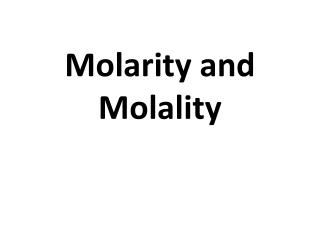Download PresentationMolarity and Molality

# Molarity and Molality - PowerPoint PPT Presentation

Molarity and Molality. Molarity ( M ). number of moles of solute (n) per liter (L) of solution Molarity ( M ) = Moles of Solute Liters of Solution Note: Moles = Mass of Solute Molar Mass of Solute.I am the owner, or an agent authorized to act on behalf of the owner, of the copyrighted work described.
Download Presentation## Molarity and Molality

Download Policy: Content on the Website is provided to you AS IS for your information and personal use and may not be sold / licensed / shared on other websites without getting consent from its author.While downloading, if for some reason you are not able to download a presentation, the publisher may have deleted the file from their server.

- - - - - - - - - - - - - - - - - - - - - - - - - - E N D - - - - - - - - - - - - - - - - - - - - - - - - - -
Presentation Transcript
1. Molarity and Molality

2. Molarity (M) number of moles of solute (n) per liter (L) of solution Molarity (M) = Moles of Solute Liters of Solution Note: Moles = Mass of Solute Molar Mass of Solute

3. 1. What is the molarity of the solution if 3.0 mole of solute is dissolved in 12.0 L solution? Given: Moles of Solute – 3.0 mole Liters of Solution – 12.0 L Formula: Molarity = Moles of Solute Liters of Solution Answer: M = Moles of Solute Liters of Solution = 3.0 mole 12.0L = 0.25 M

4. 2. What is the molarity of a 400.0 mL solution containing 8.0 g NaOH? Given: Mass of Solute – 8.0 g of NaOH Liters of Solution – 400.0 mL Formula: Molarity = Moles of Solute Liters of Solution Answer: Moles = Mass of Solute Molar Mass of Solute = 8 g of NaOH (23+16+1g/mole) = 8 g NaOH 40 g/mole = 0.2 mole

5. Given: Molarity - ? Liters of Solution – 400.0 mL Moles of Solute – 0.2 mole Formula: Molarity = Moles of Solute Liters of Solution Answer: M = Moles of Solute Liters of Solution = 0.2 mole 0.4 L = 0.5 M = 400mL X 1 L 1000mL = 0.4L

6. 3. How many grams of Ca(OH)2 are needed to prepare an 800.0 mL solution of 0.015 M concentration? Given: Molarity – 0.015 M Liters of Solution – 800.0 mL Formula: Molarity = Moles of Solute Liters of Solution Answer: Moles = (Molarity) (Liters of Sol’n) = 0.015 M (0.8 L) = 0.012 moles = 800mL X 1 L 1000mL = 0.8L

7. Given: Mass of Solute – ? Moles of Solute – 0.012 mole Molar Mass – Ca(OH)2 = [40+([16x2]+[1x2])] = 74g/mole Formula: Moles = Mass of Solute Molar Mass of Solute Answer: Moles = Mass of Solute Molar Mass of Solute Mass of Solute = (Moles) (Molar Mass of Solute) = (0.012moles) (74g/mole) = 0.89 g

8. Molality (m) as the number of moles of solute (n) per kilogram (kg) of solution Molality (m) = Moles of Solute Kilograms of Solvent Note: Moles = Mass of Solute Molar Mass of Solute

9. 1. What is the molality of a solution containing 0.46 mole of solute in 2.0 kg water? Given: Moles of Solute – 0.46 mole Kilogram of Solvent – 2.0 kg Formula: Molality = Moles of Solute Kilogram of Solvent Answer: m = Moles of Solute Kilogram of Solvent = 0.46 mole 2.0kg = 0.23 m

10. 2. What is the molality of a solution containing 75.5 g Pb(NO3)2 in 400.0 g water? Given: Mass of Solute – 75.5 g Pb(NO3)2 Molar Mass = [207+(2)+(6)] = 331 g/mole Formula: Moles = Mass of Solute Molar Mass of Solute Answer: Moles = Mass of Solute Molar Mass of Solute = 75.5 g 331 g/mole = 0.23 moles

11. Given: Molality – ? Moles of Solute – 0.23 moles Kilogram of Solvent – 400.0 g = 0.4kg Formula: m = Moles of Solute Kilogram of Solution Answer: m = Moles of Solute Kilogram of Solution = 0.23 moles 0.4 kg = 0.58 m

12. What mass of solute is needed to prepare each of the following solutions? a. 1.00 L of 0.125 M K2SO4b. 375 mL of 0.015 MNaF • Calculate the molarity of each of the following solutions:a. 12.4 g KCl in 289.2 mL solution b. 16.4 g CaCl2 in 0.614 L solution   • Calculate the molality of each of the following solutions:a. 2.89 g of NaCl dissolved in 0.159 g of water b. 1.80 molKCl in 16.0 mol of H2O